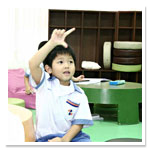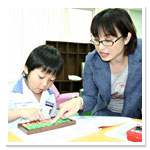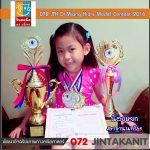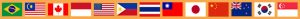# Teaching Techniques and Development ProceduresSetting up with the learning atmosphere should be emphasized on the funniness through playing games and using imagination. Then, it should be increased with good morale apart from applying for the academic contents only. Besides, this teaching is managed by the training teachers certified by the institute from using the contents of nine abacuses in kindergarten level curriculum. Indeed, the curriculum of using Abacuses with 4 : 1 is suitable for the primary students classifying into 10 levels. In addition, this curriculum is under the learning principle of calculation by using abacuses to add , subtract and let the child to begin with using of abacus imagination.  After that, she or he might be the expert in math with the brain development for both sides. Finally, he or she might begin to use Mental arithmetic to multiply , divide , add and subtract with the more complex numbers until using the imagination to calculate to be quick than using any calculator.Grade1 : Learning with the basic to use abacus for adding and subtracting

The children will be taught to know how to use nine abacuses in kindergarten level as well as practicing to use for abacus system with ratios of 4:1. Besides, it consists of the top abacus and other four bottom abacuses. Therefore, children can add and subtract the numbers through using the plural ten numeral system and the plural five numeral system. In this case, the children will gain for the real experience during their practicing of exercises at least for 100 questions. In addition, in each questions there are three to five numbers so that the children should make the analyzing by finding the answers at least for 500 times. After that, they can practice themselves by using for their left brains as the main side.

Grade 2 : Practicing by using abacuses fluently and beginning to use Mental arithmetic for adding and subtracting the numbers

In this level, children can practice for both of their right brains and left brains. Then, they should use the Mental arithmetic  by using abacuses actively. Besides, in grade 1 the children should make analyzing with calculation by using for their left brains as well as imagining about the changing as the picture. After completing from using math calculation with pictures , the children will see the results of answers. In this case, it can develop for their both sides of brain connected by Corpus Callosum. As the result, after practicing a lot their brain will be developed with having the good potential to learn new things in good wayGrade 3- 4 : Practicing Mental arithmetic until being expert in adding and subtracting before practicing in multiplying and dividing by using abacuses

expert in adding and subtracting before practicing in multiplying and dividing by using abacuses

Grade 5-7 : Practicing Mental arithmetic with increasing for good memory and mediation

In this level, children will have their activeness , quickness and accurateness to imagine for more complex numbers. Besides, they will have their better memory and mediation since they have learnt from decimal numbers , multiplying for 2 digit numbers with another 2 digit ones and dividing for 4 digit numbers with another 2 digit numbers.

Grade 8-10 : Using the high imagination to imagine for adding , subtracting , multiplying and dividing by using decimal numbers

Then, if the children have their readiness to be ready for learning in several subjects excellently , they should have their good calculating processes with good analyzing  in accurate way. As the result, they are liked the growing tree to be put the good water and fertilizer.

#### Intellectual Level

The advanced calculation with adding , subtracting , multiplying and dividing by using the decimal numbers as well as the using of negative numbers , square root 2 and square root 3.

In summary, it should take times much to develop the brain as well as using the analyzing processes with imagination. In addition, after we practice each subject a lot , it will become to be the expertness without learning only to do in easy way. On the contrast, we learn to exercise their brains for three months to six months. Then, it should learn with more numbers in the higher class level of school in order to develop their brains perfectly.```8/7 Sukhumvit 71 Road,